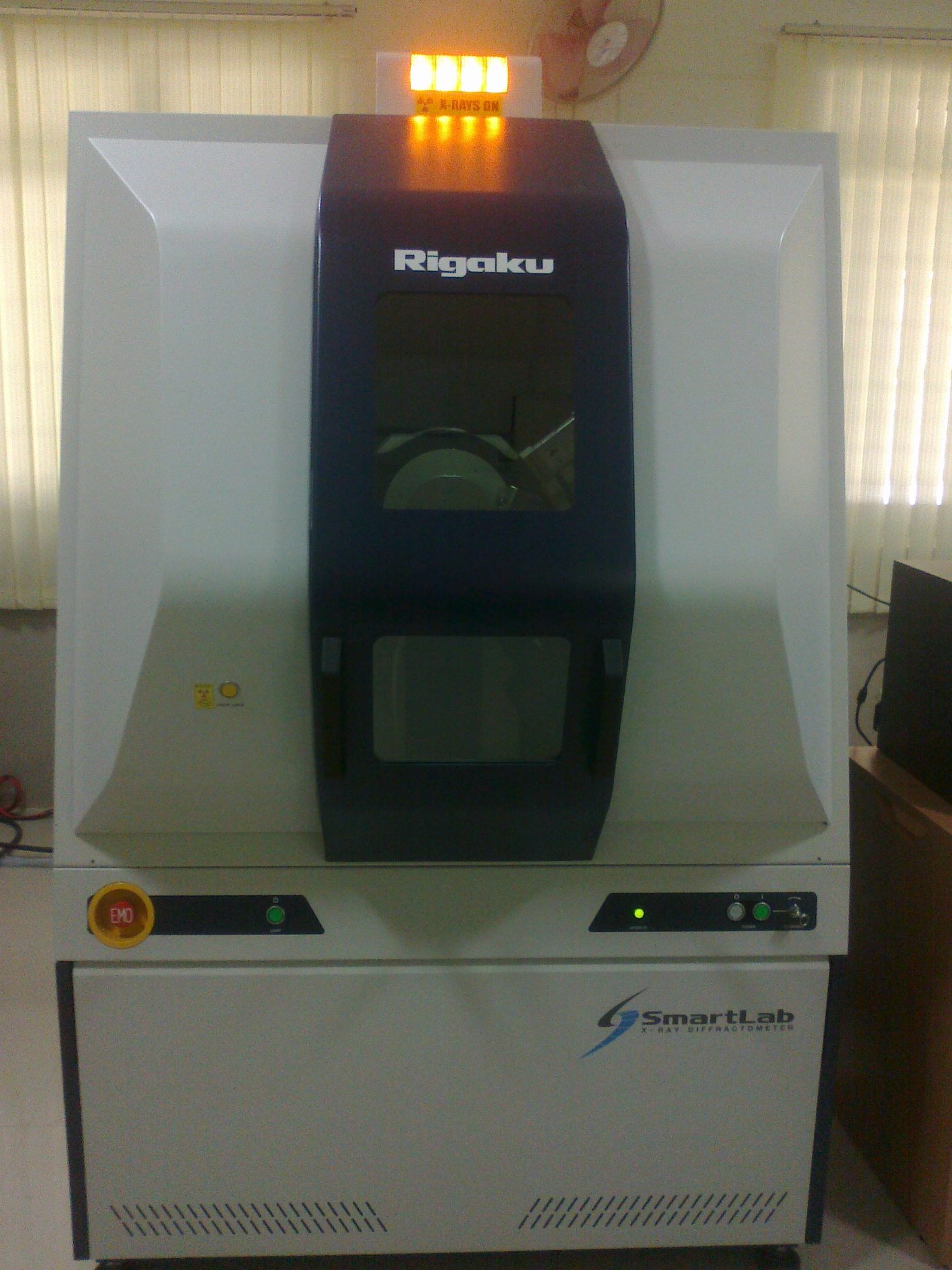# X-Ray Diffraction:### Introduction

Crystals are regular arrays of atoms. Atoms scatter X-ray waves, primarily through the atoms' electrons. An X-ray striking an electron produces secondary spherical waves emanating from the electron. This phenomenon is known as elastic scattering, and the electron is known as the scatterer. A regular array of scatterers produces a regular array of spherical waves. Although these waves cancel one another out in most directions through destructive interference, they add constructively in a few specific directions, determined by Bragg's law:

2dsinθ = nλ

Here d is the spacing between diffracting planes, is the incident angle, n is any integer, and λ is the wavelength of the beam. These specific directions appear as spots on the diffraction pattern called reflections. Thus, X-ray diffraction results from an electromagnetic wave (the X-ray) impinging on a regular array of scatterers (the repeating arrangement of atoms within the crystal).

X-rays are used to produce the diffraction pattern because their wavelength λ is typically the same order of magnitude (1–100 angstroms) as the spacing d between planes in the crystal. In principle, any wave impinging on a regular array of scatterers produces diffraction, as predicted first by Francesco Maria Grimaldi in 1665. To produce significant diffraction, the spacing between the scatterers and the wavelength of the impinging wave should be similar in size. However, visible light has too long a wavelength (typically, 5500 angstroms) to observe diffraction from crystals. Prior to the first X-ray diffraction experiments, the spacings between lattice planes in a crystal were not known with certainty.

The incoming beam (coming from upper left) causes each scatterer to re-radiate a small portion of its intensity as a spherical wave. If scatterers are arranged symmetrically with a separation d, these spherical waves will be in sync (add constructively) only in directions where their path-length difference 2d sin θ equals an integer multiple of the wavelength λ. In that case, part of the incoming beam is deflected by an angle 2θ, producing a reflection spot in the diffraction pattern